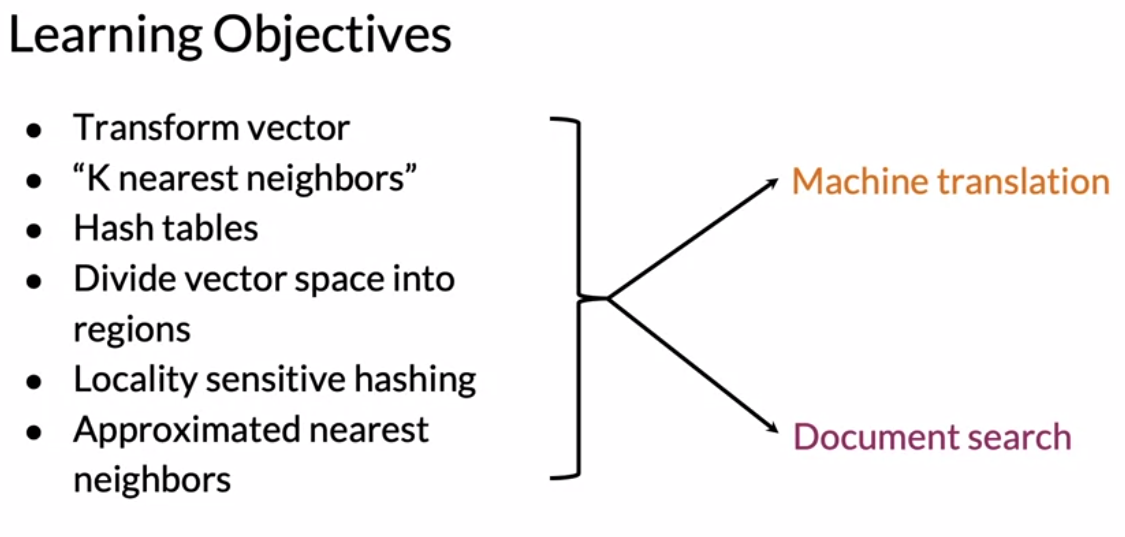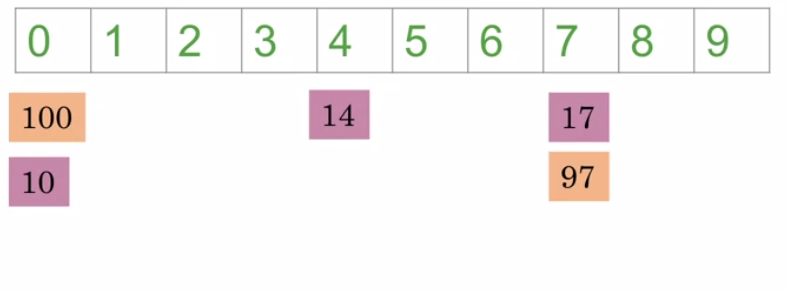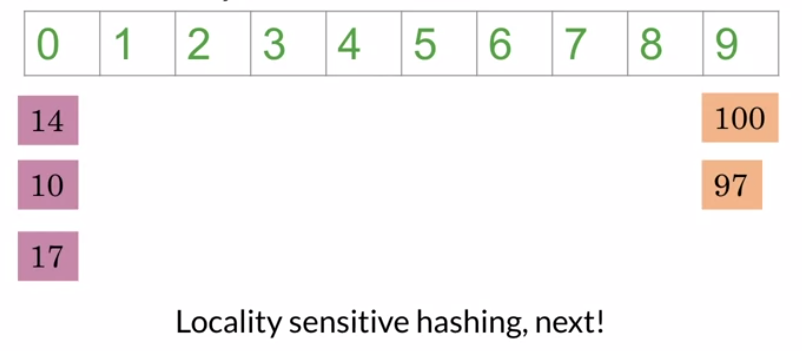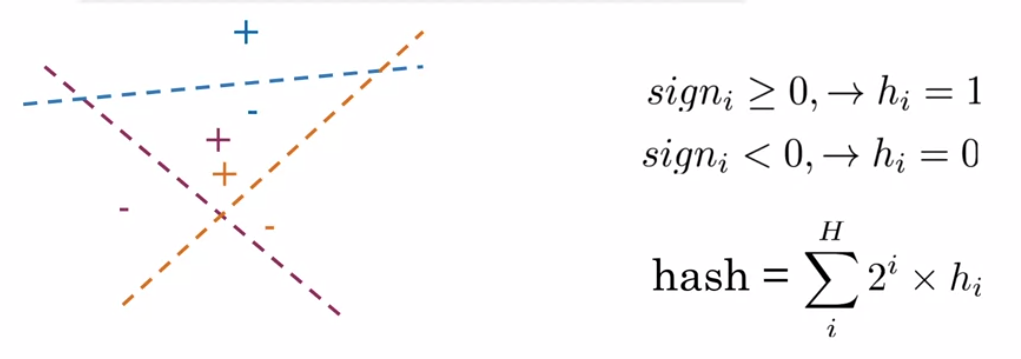## Machine Translation: An Overview## Transforming word vector

Given a set of english words X, a transformation matrix R and a desired set of french word Y the transformation

• $XR \approx Y$
• We initialize the weights R randomly and in a loop execute the following steps
• $Loss = || XR - Y||_F$
• $g = \frac{d}{dR} Loss$
• $R = R - \alpha g$

The Frobenius Norm takes all the squares of each elements of the matrix and sum them up.

• $||A||_F = \sqrt{\sum_{i=1}^{m} \sum_{j=1}^{n} |a_{ij}|^2}$

To simplify we can take the norm squared, thus:

• $||A||^2_F = \sum_{i=1}^{m} \sum_{j=1}^{n} |a_{ij}|^2$

Gradient:

• $g = \frac{d}{dR} Loss = \frac{2}{m} (X^T (XR-Y))$

## Hash tables and hash functions

Hash might skip other proprieties of the itens being hashed.To ensure that the itens are hashed accordingly we will use Locality sensitive hashing.## Locality sensitive hashing

With multiple plans we can use a binary encoding to give the hash of the position given by the position.